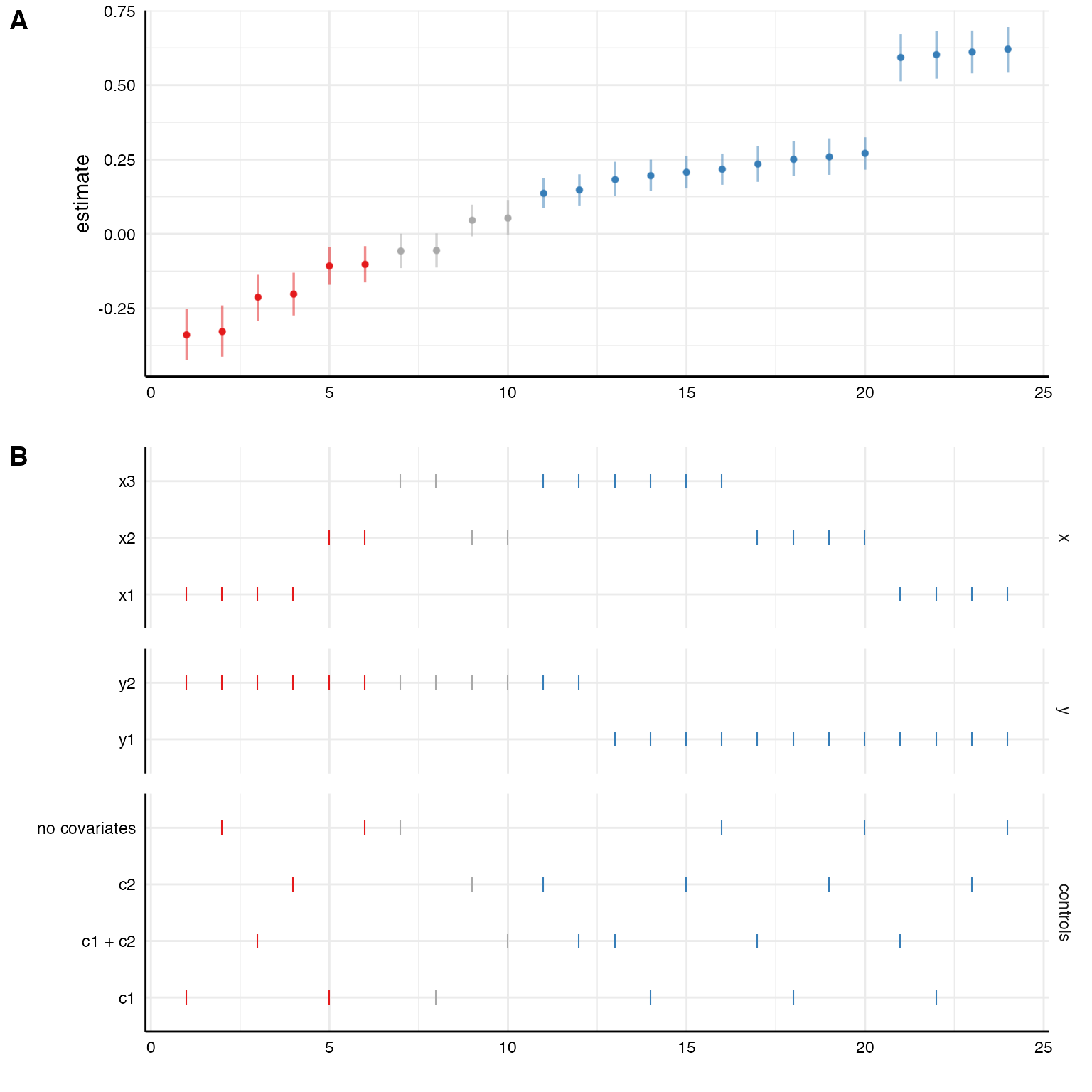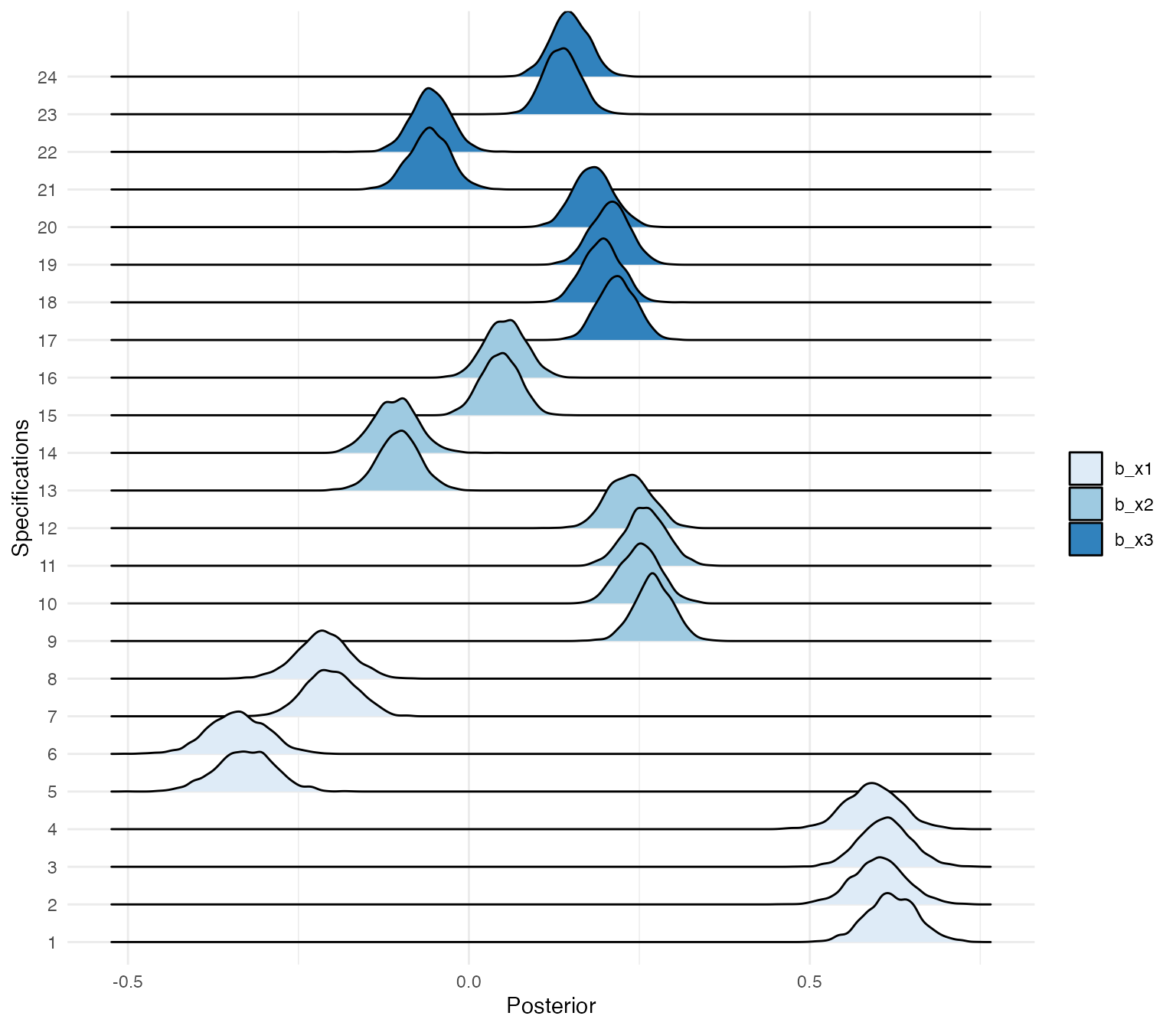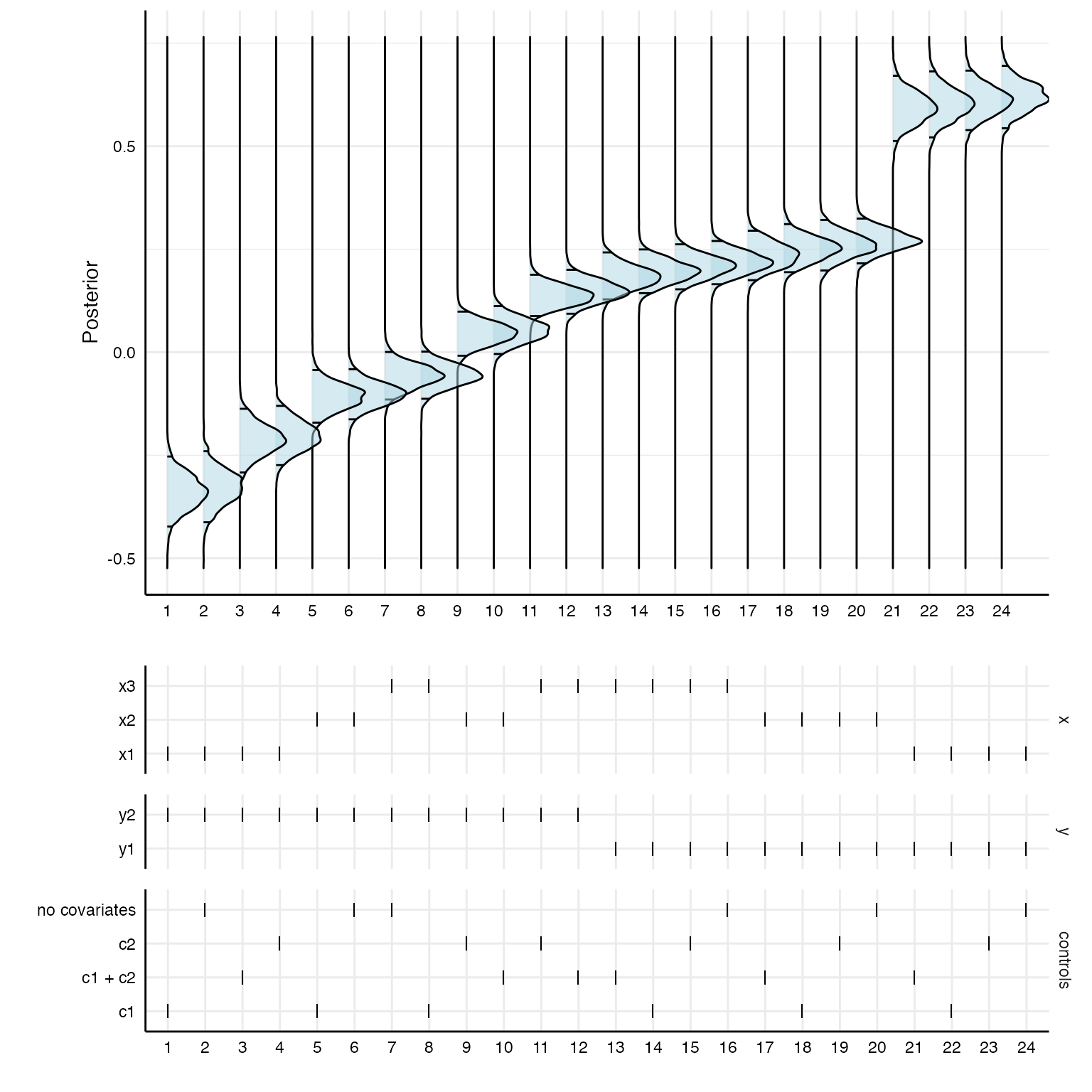This vignette shows how to incorporate Bayesian modeling using the package brms in specr. When we fit a model with brms, the package actually calls Rstan in the background, which, in turn, is an R interface to the statistical programming language Stan. Stan is built in the programming language C++ and models have to be compiled using C++ to be run. This is all taken care of by brms, so you just need to run brm(…) to fit any model. brms uses a syntax for specifying model formulae that is based on the syntax of the commonly known lme4 package (for multilevel modeling). The comparatively easy syntax of brms is then converted into Stan code automatically.

That said, since the models have to be compiled in C++, you need to set up your computer so that it can actually use C++. This has to be done only once, before installing brms. Unfortunately, as almost always, how you install C++ depends on your operating system. Check this vignette to if you have problems installing brms.

We are also loading the library furrr as we want to parallelize the computations.

library(tidyverse)
library(specr)
library(brms)
library(broom.mixed)
library(ggridges)

## Setting up custom functions

Although you can simply pass the function brm to setup and run specr() with workers = 1 without any specific custum function, it usually make sense to set up some custom model fitting and extraction functions to make sure specr() does exactly what we want.

For example, I would suggest to create a custom model fitting function and specify relevant parameters for the Bayesian models. Here, I switch off parallel processing as I want to do this via specr() (i.e., setting cores = 1 in brm). I also reduce the number of chains to 1 to speed up the fitting process (but we can bump this up if we want). This custom function also allows me to load brms itself and broom.mixed, which provides the tidy function to extract parameters from the brm.fit object.

Furthermore, I also create a custom fit extract function. Most importantly, I add the full brms.fit object to the glance output. This way, we can later access the entire object in order to e.g., extract posterior distributions or other aspects of the Bayesian models.

# Customized function, not necessary if standard values are to be used
brm_new <- function(formula, data) {
brm(formula, data,
silent = 2,    # Don't print progress
refresh = 0,   # Remove message (not necessary, but nicer in the output)
iter = 1000)   # I just set this here to reduce computing time, can be higher, of course
}

# New fun2, fit extract function
glance_brm <- function(x) {
fit2 <- broom::glance(x)
fit2$full_model <- list(x) # add full model return(fit2) } # Setting up specifications specs <- setup(data = example_data, x = c("x1", "x2", "x3"), y = c("y1", "y2"), model = "brm_new", controls = c("c1", "c2"), fun2 = glance_brm) # Check specs summary(specs) #> Setup for the Specification Curve Analysis #> ------------------------------------------- #> Class: specr.setup -- version: 1.0.0 #> Number of specifications: 24 #> #> Specifications: #> #> Independent variable: x1, x2, x3 #> Dependent variable: y1, y2 #> Models: brm_new #> Covariates: no covariates, c1, c2, c1 + c2 #> Subsets analyses: all #> #> Function used to extract parameters: #> #> function(x) broom::tidy(x, conf.int = TRUE) #> <environment: 0x7f9c7371bad0> #> #> #> Head of specifications table (first 6 rows): #> # A tibble: 6 × 6 #> x y model controls subsets formula #> <chr> <chr> <chr> <chr> <chr> <glue> #> 1 x1 y1 brm_new no covariates all y1 ~ x1 + 1 #> 2 x1 y1 brm_new c1 all y1 ~ x1 + c1 #> 3 x1 y1 brm_new c2 all y1 ~ x1 + c2 #> 4 x1 y1 brm_new c1 + c2 all y1 ~ x1 + c1 + c2 #> 5 x1 y2 brm_new no covariates all y2 ~ x1 + 1 #> 6 x1 y2 brm_new c1 all y2 ~ x1 + c1 ## Estimating the models Because we are estimating 24 Bayesian models, this may take a while, but brms automatically parallelizes the computations. results <- specr(specs) Now we can again summarize and plot our results. summary(results) #> Results of the specification curve analysis #> ------------------- #> Technical details: #> #> Class: specr.object -- version: 1.0.0 #> Cores used: 1 #> Duration of fitting process: 9.25 sec elapsed #> Number of specifications: 24 #> #> Descriptive summary of the specification curve: #> #> median mad min max q25 q75 #> 0.16 0.25 -0.34 0.62 -0.07 0.25 #> #> Descriptive summary of sample sizes: #> #> median min max #> 1000 1000 1000 #> #> Head of the specification results (first 6 rows): #> #> # A tibble: 6 × 18 #> x y model controls subsets formula effect component group estimate std.error #> <chr> <chr> <chr> <chr> <chr> <glue> <chr> <chr> <chr> <dbl> <dbl> #> 1 x1 y1 brm_new no covariates all y1 ~ x1 + 1 fixed cond <NA> 0.62 0.04 #> 2 x1 y1 brm_new c1 all y1 ~ x1 + c1 fixed cond <NA> 0.6 0.04 #> 3 x1 y1 brm_new c2 all y1 ~ x1 + c2 fixed cond <NA> 0.61 0.04 #> 4 x1 y1 brm_new c1 + c2 all y1 ~ x1 + c… fixed cond <NA> 0.59 0.04 #> 5 x1 y2 brm_new no covariates all y2 ~ x1 + 1 fixed cond <NA> -0.33 0.04 #> 6 x1 y2 brm_new c1 all y2 ~ x1 + c1 fixed cond <NA> -0.34 0.04 #> # … with 7 more variable: conf.low <dbl>, conf.high <dbl>, fit_algorithm <chr>, fit_pss <dbl> #> # fit_nobs <dbl>, fit_sigma <dbl>, fit_full_model <list> plot(results, choices = c("x", "y", "controls"))Again, the coefficients are median estimates from the posterior and their respective 95% HDIs. ## Inspecting specific models Because we kept the entire brms models in our result data set, we can explore specific models using the pull function. # Pull entire models from the listed vector models <- results %>% as_tibble %>% pull(fit_full_model) # Summarize e.g., the first model summary(models[]) #> Family: gaussian #> Links: mu = identity; sigma = identity #> Formula: y1 ~ x1 + 1 #> Data: data (Number of observations: 1000) #> Draws: 4 chains, each with iter = 1000; warmup = 500; thin = 1; #> total post-warmup draws = 2000 #> #> Population-Level Effects: #> Estimate Est.Error l-95% CI u-95% CI Rhat Bulk_ESS Tail_ESS #> Intercept -1.10 0.04 -1.17 -1.02 1.00 1915 1356 #> x1 0.62 0.04 0.55 0.69 1.00 1900 1631 #> #> Family Specific Parameters: #> Estimate Est.Error l-95% CI u-95% CI Rhat Bulk_ESS Tail_ESS #> sigma 1.14 0.03 1.09 1.18 1.00 2903 1542 #> #> Draws were sampled using sampling(NUTS). For each parameter, Bulk_ESS #> and Tail_ESS are effective sample size measures, and Rhat is the potential #> scale reduction factor on split chains (at convergence, Rhat = 1). From these model fit objects, we can also extract posterior distributions for the parameters of interest and stort them in one data frame. posteriors <- results %>% as_tibble %>% pull(fit_full_model) %>% map(function(x) as_tibble(as_draws_df(x))[, 2] %>% gather(key, value)) %>% bind_rows(., .id = "id") # First 10 draws from the first specification for predictor x2 head(posteriors, n = 10) #> # A tibble: 10 × 3 #> id key value #> <chr> <chr> <dbl> #> 1 1 b_x1 0.642 #> 2 1 b_x1 0.655 #> 3 1 b_x1 0.647 #> 4 1 b_x1 0.650 #> 5 1 b_x1 0.611 #> 6 1 b_x1 0.736 #> 7 1 b_x1 0.565 #> 8 1 b_x1 0.624 #> 9 1 b_x1 0.602 #> 10 1 b_x1 0.611 ## Plotting posterior distributions for all specifications Using the ggridges package, we can plot the posterior distributions of the different specifications. posteriors %>% mutate(id = factor(id, levels = 1:24)) %>% ggplot(aes(x = value, y = id, fill = key)) + geom_density_ridges() + scale_fill_brewer(palette = "Blues") + theme_minimal()+ labs(x = "Posterior", y = "Specifications", fill = "")Of course, the posterior distributions are not sorted in any way. With a bit of data wrangling, we can create a specification curve consisting of posterior distributions. # First panel p1 <- results %>% as_tibble %>% mutate(id = as.character(1:nrow(.))) %>% arrange(estimate) %>% mutate(specifications = factor(as.character(1:nrow(.)), levels = c(1:24))) %>% left_join(posteriors) %>% ggplot(aes(x = value, y = specifications)) + stat_density_ridges(quantile_lines = TRUE, quantiles = c(0.025, 0.975), alpha = .5, fill = "lightblue", color = "black") + coord_flip() + theme_minimal() + labs(x = "Posterior", y = "") + theme(strip.text = element_blank(), axis.line = element_line("black", size = .5), legend.position = "none", panel.spacing = unit(.75, "lines"), axis.text = element_text(colour = "black")) # Second panel p2 <- results %>% as_tibble %>% arrange(estimate) %>% mutate(specifications = factor(as.character(1:nrow(.)), levels = c(1:24))) %>% gather(key, value, c("x", "y", "controls")) %>% mutate(key = factor(key, levels = c("x", "y", "controls"))) %>% ggplot() + geom_point(aes(x = specifications, y = value), shape = 124, size = 3.35) + theme_minimal() + facet_grid(.data$key~1, scales = "free_y", space = "free_y") +
theme(
axis.line = element_line("black", size = .5),
legend.position = "none",
panel.spacing = unit(.75, "lines"),
axis.text = element_text(colour = "black"),
strip.text.x = element_blank()) +
labs(x = "", y = "")

# Combine
plot_grid(p1, p2,
ncol = 1,
align = "hv",
axis = "tblr",
rel_heights = c(3, 2))